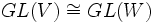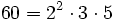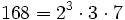# General linear group over a field

(Redirected from General linear group)
Jump to: navigation, search
This article defines a natural number-parametrized system of algebraic matrix groups. In other words, for every field and every natural number, we get a matrix group defined by a system of algebraic equations. The definition may also generalize to arbitrary commutative unital rings, though the default usage of the term is over fields.
View other linear algebraic groups|View other affine algebraic groups

This article is about the unit group (group of invertible elements) in the following ring/monoid: matrix ring

## Definition

### In terms of dimension (finite-dimensional case)

Let$n$ be a natural number and$k$ a field. The general linear group of degree$n$ over$k$, denoted$GL(n,k)$, is defined in the following equivalent ways:

•$GL(n,k)$ is the group of all invertible$k$-linear maps from the vector space$k^n$ to itself, under composition. In other words, it is the group of automorphisms of$k^n$ as a$k$-vector space.
•$GL(n,k)$ is the group of all invertible$n \times n$ matrices with entries over$k$

### In terms of vector spaces

Let$V$ be a$k$-vector space (which may be finite or infinite-dimensional). The general linear group over$V$, denoted$GL(V)$, is the group of all vector space automorphisms from$V$ to itself.

Note that when$V = k^n$, this reduces to the definition$GL(n,k)$. Further, since$GL(V) \cong GL(W)$ for$V \cong W$, and since any two vector spaces of the same dimension are isomorphic, the$GL(n,k)$s cover all general linear groups corresponding to finite-dimensional vector spaces.

## More general versions

Some more general versions occur where we replace field by more general constructs:

Replacement of field Corresponding analogue of general linear group
division ring general linear group over a division ring
commutative unital ring general linear group over a commutative unital ring
unital ring general linear group over a unital ring

## As a group with additional structure

### As an algebraic group

Further information: general linear group over a field as an algebraic group

For any field$k$, the general linear group$GL(n,k)$ has the natural structure of an algebraic group. It can be viewed as a Zariski-open subvariety in$k^{n^2}$ given by the condition that the determinant function does not vanish.

### As a Lie group

In the particular cases of the real numbers, complex numbers, and other topological fields, the general linear group acquires the structure of a Lie group over that field.

## As a map

### As a functor from fields to groups

If we fix$n$, we can think of$GL_n$ as a functor from the category of fields to the category of groups.

### As an IAPS

Further information: GL IAPS

For a fixed field$k$, the general linear groups$GL(n,k)$ form an IAPS of groups parametrized by$n$. In other words, we naturally have concatenation maps:$\Phi_{m,n}: GL(m,k) \times GL(n,k) \to GL(m+n,k)$

This map takes a matrix$A$ of order$m$ and a matrix$B$ of order$n$ and putputs the block diagonal matrix with blocks$A$ and$B$.

### As a functor from fields to IAPSes

If we fix neither$n$ nor$k$, we can view$GL$ as a functor from fields to the category of IAPSes of groups.

## Subgroups

The general linear group has a number of important subgroups. Some of them are listed below:

• Orthogonal group is the subgroup comprising those matrices$A$ such that$AA^t$ is the identity matrix. Further information: Orthogonal group in general linear group
• Special linear group is the subgroup comprising those matrices with determinant 1. Further information: Special linear group in general linear group
• Symplectic group is the subgroup comprising matrices$A$ such that$APA^t = P$ where$P$ is a certain fixed matrix. Further information: Symplectic group in general linear group
• Special orthogonal group is the intersection of the orthogonal group and the special linear group
• Orthogonal similitude group is the group of matrices$A$ such that$AA^t$ is a scalar matrix
• Symmetric group which is the group of permutation matrices, embedded in the general linear group

All of these, except the orthogonal similtude group, form sub-IAPSes.

## Quotients

### Inner automorphism group

The inner automorphism group of this group, viz the quotient group by its center, is abstractly isomorphic to: projective general linear group

## Supergroups

Some important groups in which the general linear group is contained:

## Particular cases

### Finite fields

Here are some general facts:

• Multiplicative group of a finite field is cyclic
• Special linear group is quasisimple for$n \ge 2$, except the case that$n = 2$ and$k$ has two or three elements. Thus, all the corresponding general linear groups have a simple non-abelian subquotient. In the case that the field has characteristic two, the general linear group coincides with the special linear group, and it is centerless, so it turns out to be a simple non-abelian group itself.
Size of field Order of matrices Common name for the general linear group Order of group Comment
2 1 Trivial group$1$ Trivial
3 1 Cyclic group:Z2$2$ group of prime order
4 1 Cyclic group:Z3$3$ group of prime order
5 1 Cyclic group:Z4$4 = 2^2$ cyclic group
2 2 Symmetric group:S3$6 = 2 \cdot 3$ supersolvable but not nilpotent
3 2 General linear group:GL(2,3)$48 = 2^4 \cdot 3$ solvable but not supersolvable
4 2 Alternating group:A5$60 = 2^2 \cdot 3 \cdot 5$ simple non-abelian group
5 2 General linear group:GL(2,5)$480 = 2^5 \cdot 3 \cdot 5$ not solvable, has a simple non-abelian subquotient.
2 3 General linear group:GL(3,2)$168 = 2^3 \cdot 3 \cdot 7$ simple non-abelian group

## GAP implementation

The general linear group can be implemented using the GAP function GeneralLinearGroup that can be invoked as either GeneralLinearGroup or GL. It takes two arguments, the first being the degree (i.e., the order of matrices) and the second either a ring or a prime power (for which we consider the corresponding field). For instance, for the general linear group of degree two over the field of three elements:

G := GL(2,3);